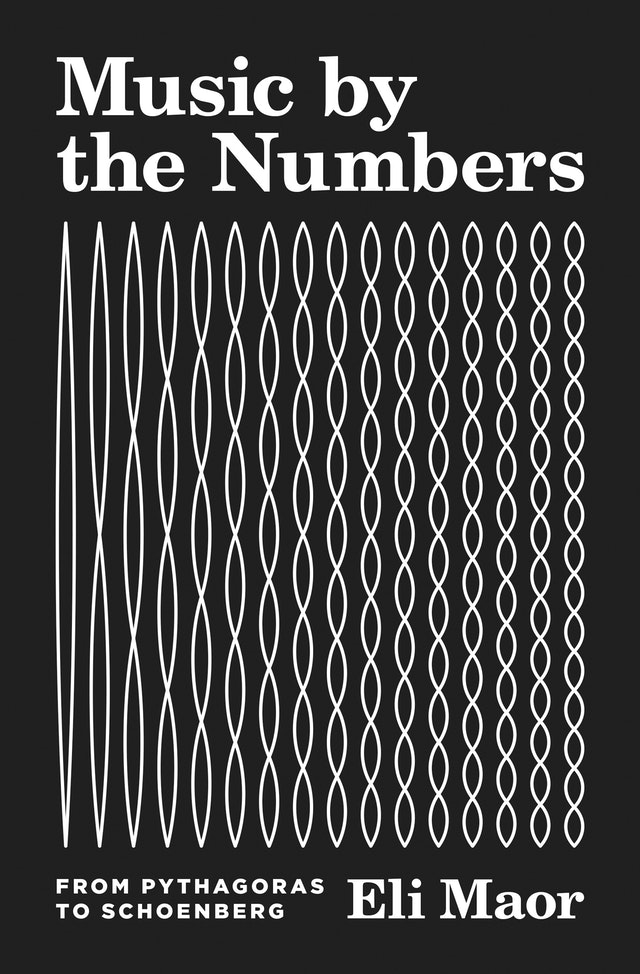The book Music by the Numbers by Eli Maor is a light introduction to the many contributions to the music theory from the scientific community. An unexpected number of well-known scientists actually stepped onto the stage of the music history as well.

This blog posts will summarize a short (and incomplete) bullet list of items that are presented in the book.

# Pythagoras and “numbers rule the universe”

Pythagoras of Samos (ca. 585–500 BCE) built an instrument called monochord, which is a single string attached to a soundboard with a numerical scale. He found that a pitch and one octave above it has a ratio of 1:2 (or 2:1 in terms of string lengths).

He further used his “numbers rule the universe” philosophy and generalized that finding to have found the octaves (1:2), the fifths (2:3), the fourth (3:4). He further generalized that to the Pythagoras diatonic scale, which is

$\frac{9}{8} \frac{9}{8} \frac{256}{243} \frac{9}{8} \frac{9}{8} \frac{9}{8} \frac{256}{243}.$

Simple as it is, it’s often critiqued as it is slightly off when compared to today’s equal temperament tuning. Also, it does not quite deal with multiple octaves, as the fractional numbers will go off even further.

# Mersenne measured the frequencies

Marin Mersenne (1588–1648) is mostly known for Mersenne primes, i.e., primes of the shape $$M_n = 2^n - 1$$. He is also the pioneer to actually measure the frequencies of sounds.

# Sauveur discovered the overtones

Joseph Sauveur (1653–1716) found that plucking a string at 1/2 of its length, 1/3 and 2/3 of its length, 1/4, 2/4/ and 3/4 of its length, … will give harmonic tones. These are overtones and altogether can form a musical instrument’s unique timbre. The infinite diverging series $$1+\frac{1}{2}+\frac{1}{3}+\frac{1}{4}+\frac{1}{5}+\dots$$ in math is also called a harmonic series.

# Fourier series and sounds

Jean Baptiste Joseph Fourier (1768–1830) discovered that every periodic function with peiord $$2\pi$$, with certain restrictions, can be rewritten as the following:

$f(x) = \frac{a_0}{2}+\sum_{n=1}^\infty(a_n \cos nx + b_n \sin nx).$

Since every periodic vibration produces a music sound, it can be broken into the harmonic components using Fourier series.

# Brief history of equal temperament tuning

• Aristoxenus of Tarentum (fl. 335 BCE), a pupil of Aristotle, rejected Pythagoras’s ideal of “numbers rule the universe”, but insisted that intervals should be judged by the ear alone.
• Vincenzo Galilei revived Aristoxenus’s idea after two thousand years, suggesting that dividing an octave of 12 equal semitones, each having the ratio 18:17, making the octave slightly flat [$$(\frac{18}{17})^{12}\sim 1.9856$$].
• Zhu Zaiyu (1536–1611), chinese prince in Ming Dynasty, wrote several treatises on music theory in which he proposed an equal temperament.
• Simon Stevin (1548–1620), Flemish mathematician, insisted the fifth should have the ratio $$(\sqrt{2})^7 \sim 1.4983$$.
• Marin Mersenne in 1636 suggested a semitone to have the ratio $$\sqrt{\frac{2}{3-\sqrt{2}}}\sim 1.0597$$.
• Johann Sebastian Bach (1685–1750) composed The Well-Tempered Clavier and popularized the use of equal temperament scale.

# Tuning forks

• John Shore, and English trumpeter and lute player, invented tuning fork in 1711.
• Some tuning fork settings (note A above middle C)
• John Shore: 423.5 Hz
• Rudolph König: 435 Hz
• Handel (1751): 422.5 Hz
• Mozart (1780): 421.6 Hz
• Current standard: 440 Hz. Adopted at a congress of physicists in Stuttgard, Germany in 1834. International-wide adoption until 1939.

# Metronomes

Invented by Dietrich Nikolaus Winkel in 1814. Stolen and manufactured (thus popularized) by Johann Nepomuk Mälzel (1772–1838).

# Schoenberg and relativistic music

A method of composing with 12 tones which are related only with one another. The select 12 notes form a tone row, which replaces the traditional theme. Prescribed rules are used to change the notes, such as play backward, upside down, or in retrograde inversion. The row can then be developed in a variety of ways, leading to different music pieces.